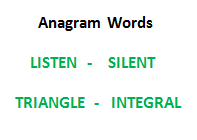Open In App

# String Anagram in C

An anagram of a string is another string that is formed by the rearrangement of the same set of characters. For example, “abcd” and “dabc” are anagrams of each other.

In this article, we will learn how to check whether two strings are anagrams of each other in the C programming language.The idea is to count the frequency of each character in both strings. If they match, the two strings are anagrams of each other. There might be a case where the length of two strings is not equal so we also need to check if there are any remaining characters in either str1 or str2 before comparing the frequency of each character of both strings.

## Algorithm

1. Initialize two arrays named count1 and count2 of size 256 elements with each element 0.
2. Start a loop to traverse string1 and string2 till any of them reaches NULL.
3. For each character in the string, increment the value of the count element present at the index corresponding to the ASCII value of the character.
4. After the loop, if string1[i] and string2[i] are not NULL, return false.
5. Else, compare the count of each character of string1 and string2.
1. If the count1 and count2 are same, return true.
2. Else, return false.

## C

 `// C program to check if two strings``// are anagrams of each other``#include ``#define NO_OF_CHARS 256`` ` `/* Function to check whether two``   ``strings are anagram of each other */``int` `areAnagram(``char``* str1, ``char``* str2)``{``    ``// Create 2 count arrays and initialize``    ``// all values as 0``    ``int` `count1[NO_OF_CHARS] = { 0 };``    ``int` `count2[NO_OF_CHARS] = { 0 };``    ``int` `i;`` ` `    ``// For each character in input strings,``    ``// increment count in the corresponding``    ``// count array``    ``for` `(i = 0; str1[i] && str2[i]; i++) {``        ``count1[str1[i]]++;``        ``count2[str2[i]]++;``    ``}`` ` `    ``// If both strings are of different length.``    ``// Removing this condition will make the``    ``// program fail for strings like "aaca"``    ``// and "aca"``    ``if` `(str1[i] || str2[i])``        ``return` `0;`` ` `    ``// Compare count arrays``    ``for` `(i = 0; i < NO_OF_CHARS; i++)``        ``if` `(count1[i] != count2[i])``            ``return` `0;`` ` `    ``return` `1;``}`` ` `// Driver code``int` `main()``{``    ``char` `str1[] = ``"geeksforgeeks"``;``    ``char` `str2[] = ``"forgeeksgeeks"``;`` ` `    ``// Function Call``    ``if` `(areAnagram(str1, str2))``        ``printf``(``"The two strings are anagram of each other"``);``    ``else``        ``printf``(``"The two strings are not anagram of each "``               ``"other"``);``    ``return` `0;``}`` ` `// This code is contributed by Pushpesh Raj.`

Output

`The two strings are anagram of each other`

### Complexity Analysis

• Time Complexity: O(n)
• Auxiliary space: O(n)

Please refer complete article on Check whether two strings are anagram of each other for more details!